# How to SUM Values Between Two Dates (using SUMIFS formula)

When you’re working with datasets that have dates, you would often find yourself trying to do calculations based on the dates.

For example, if you have the sales data for a month, you may want to know the total sales that have happened between two given dates or the total sales made on weekends vs weekdays.

One of the ways to quickly get these answers is by using the SUMIFS function.

SUMIFS (as the name suggests), allows you to sum a range based on criteria. Within SUMIFs, you can specify multiple conditions and it will sum only those cells/values that meet all the conditions.

In this tutorial, I will show you how to sum values between two dates using the SUMIFS function.

So let’s get started!

This Tutorial Covers:

## SUM all values between two dates

Suppose you have a dataset as shown below and you want to know the sales that have come in during 1-Jan-2020 and 31-Jan-2020 (I am using the DD-MM-YYYY format for the date here)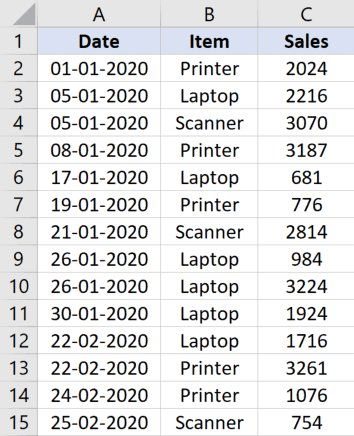Below is the formula that will give you the sum of sales between these two dates:

`=SUMIFS(C2:C15,A2:A15,">=1-1-2020",\$A\$2:\$A\$15,"<=31-01-2020")`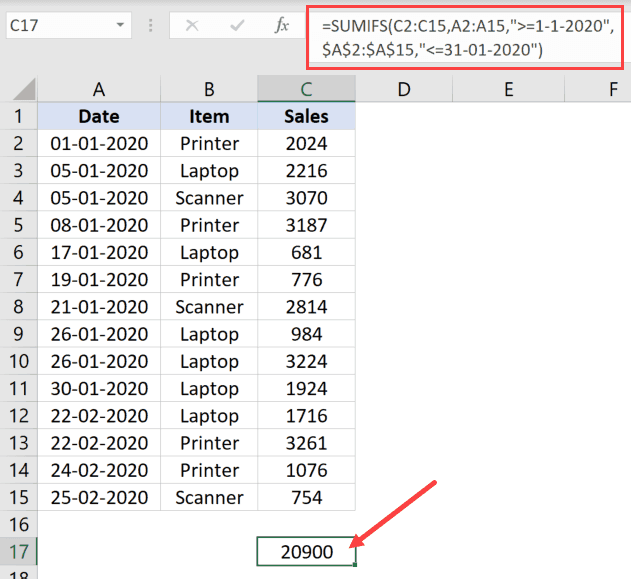The above SUMIF function uses five arguments (this can change based on the number of conditions you have):

• The first argument (C2:C15) is the range that has the values that we want to add
• The second and third argument is for the first condition, which is that the date should be more than or equal to 01-01-2020. For each condition, you need to specify the criteria range and the criteria
• The fourth and fifth arguments are for the second condition – criteria and the criteria range.

In the above formula, I have hard coded the dates. You can also have the dates in a cell and use the cell reference instead. Also, when your condition involves using an operator (such as = or <>), you need to put the operator in the double quotes.

Note: In the formula, you can use any valid date format in the formula, For example, in the cells, I have used the date as 01-01-2020, but in the formula, I can use any format that still refers to this date. For example, I can use 01 Jan, 2020 or 01 January 2020 or 1-Jan-2020. As long as the date format is valid, Excel will be able to use it to calculate the sum between the two given dates.
For example, if you have the start date and end date in cells (as shown below), you can use the following formula to get the sum of sales in the given date range.
`=SUMIFS(C2:C15,A2:A15,">="&F1,\$A\$2:\$A\$15,"<="&F2)`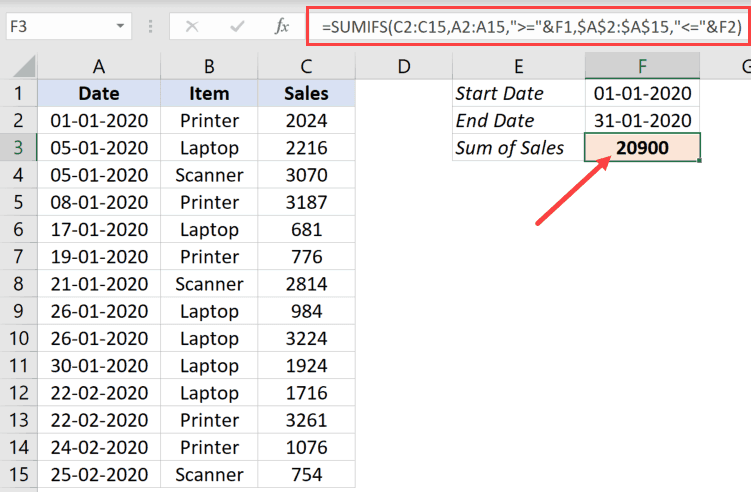Remember that the operator needs to be in double quotes, and the cell reference needs to be out of double quotes.

`Also read: Calculate Days Between Two Dates Excel`

## SUM all values between two dates for a specific product

Since the SUMIFS function allows you to use multiple conditions, you can also add more criteria in the same formula.

For example, suppose you have the same dataset (as shown below), but this time, you want to know the total sales of Printers that happened between the two given dates (01 Jan and 31 Jan).You can do that by adding another condition to the formula where apart from checking for the date, it also checks whether the product is Printer or not. It will then give you the result that matches all the given conditions

Below is the formula that will do this:

`=SUMIFS(C2:C15,A2:A15,">=1-1-2020",\$A\$2:\$A\$15,"<=31-01-2020",\$B\$2:\$B\$15,"Printer")`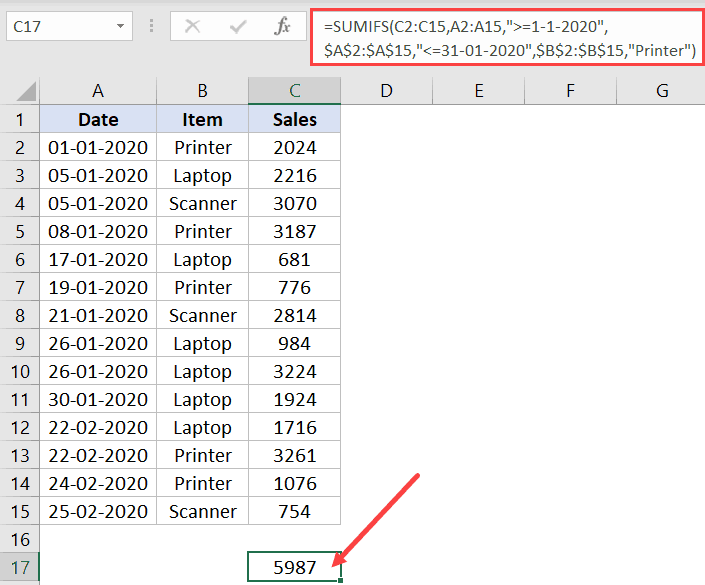The above formula checks for the dates as well as whether the product is a Printer or not. It would only sum a value when all three conditions are met.

Similarly, you can also get the sum of values between dates where you want to exclude a specific product.

For example, if you want to sum values between 1 and 31 Jan for all the product except the Scanner, then you can use the below formula:

` =SUMIFS(C2:C15,A2:A15,">=1-1-2020",\$A\$2:\$A\$15,"<=31-01-2020",\$B\$2:\$B\$15,"<>Scanner")`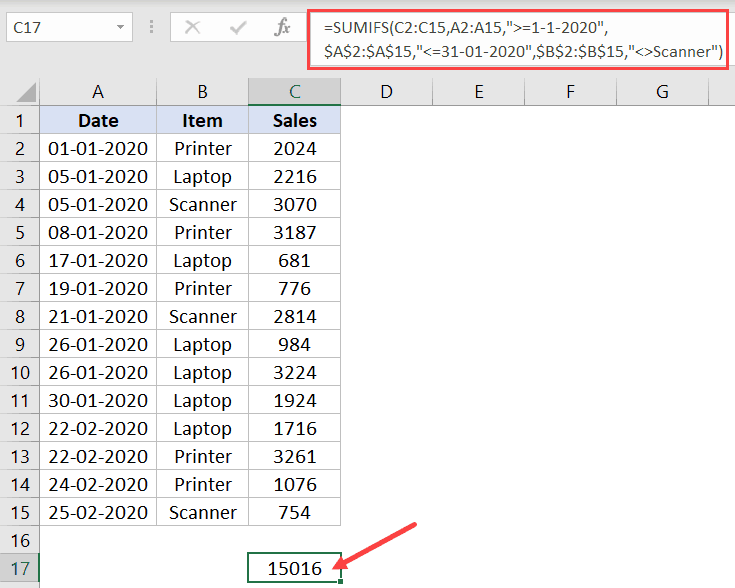In the above formula, I have used the not-equal-to-operator (<>) to exclude getting the values for Scanner in the result.

And just to reiterate, I have hard-coded the data values in the formula, but if you have these dates in a cell, you can refer to that cell in the formula.

These are some examples where you can sum values between two dates, and you can tweak the formula to add more conditions if you want.

Hope you found this tutorial useful.

You may also like the following Excel tutorials:FREE EXCEL BOOK

## Get 51 Excel Tips Ebook to skyrocket your productivity and get work done fasterHello there! I'm Sumit Bansal, founder of trumpexcel.com and an Excel MVP. I started this website in 2013 with a simple goal: to share my love for Excel through easy to follow tips, tutorials and videos. I'm here to help you get the best out of MS Excel to save time and boost your productivity.